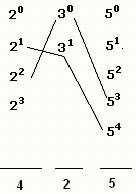How do we find divisors of a number? For example, how do we calculate the number of divisors of 900?

Answer: 900 = 22 × 32 × 52. Therefore, any number that is a factor of 900 can have powers of 2 equal to 20, 21 or 22. Similarly, for 3, the powers can be 30, 31, or 32 and for 5 they will be 50, 51, or 52. Writing the powers in a line we have-Now any combination of a power of 2, a power of 3, and a power of 5 will give us a divisor. For example, in the figure, 21 × 32 × 51 will be a divisor of 900. As we can select a power of 2 in 3 ways, a power of 3 in 3 ways, and a power of 5 in 3 ways, the total number of combinations will be 3 × 3 × 3 = 27. Therefore, the number of divisors of 900 is 27.

Find the number of divisors of 15000.

Answer: 15000 = 23 × 3 × 54. The powers of various prime factors in a divisor can be:

2 – 20, 21, 22, or 23.

3 – 30 or 31

5 – 50, 51, 52, 53, or 54.Again, the total number of combinations- and hence the total number of divisors- will be 4 × 2 × 5 = 40.

Find the number of odd divisors (divisors which are odd numbers) of 15000.

Answer: Odd numbers are not divisible by 2, and therefore in an odd divisor, there will not be a power of 2. Therefore, we will only consider powers of 3 and 5. Hence, the total number of combinations- and hence the total number of divisors- in this case, will be 2 × 5 = 10

Find the number of even divisors of 15000.

Number of even divisors = Total number of divisors – number of odd divisors

= 40 – 10 = 30.

Find the number of divisors of 15000 that are perfect squares.

Answer: In a perfect square, the power of every prime factor is even. Therefore, we will only consider those divisors of 15000 in which powers of prime factors are even. In other words, we will only consider even powers of prime factors. We write down only the even powers of prime factorsWe can see that now the number of possible combinations = 2 × 1 × 3 = 6.

Therefore, 6 divisors of 15000 are perfect squares.

Find the number of divisors of 7! that are odd.

Answer: This question is same as the earlier question except that now we will first have to do prime factorization of 7!. For that, we will have to find powers of the prime factors in 7!. The prime factors present in 7! will be 2, 3, 5 and 7. Their respective powers are:Therefore, 7! = 24 × 32 × 5 × 7. To find odd divisors of 7!, we ignore the powers of 2 and then calculate the number of combinations of powers of 3, 5, and 7. The number of combinations = 3 × 2 × 2 = 12. Therefore, there are 12 odd divisors of 7!.

http://tathagat.mba/forums/number-system/divisors-2/

http://tathagatclasses.com/cbt/

• Nice instruction, thanks

By LouisHayes , 3 months ago

• In a test match in cricket, the scores of Rohit and Vir...

By nhuadovan , 12 months ago

• The 111th digit of the series 149162536496481........ i...

By nhuadovan , 12 months ago

• how many perfect squares of natural numbers between 300...

By nhuadovan , 12 months ago

• Find the number of ways to distribute 2n different ball...

By nhuadovan , 12 months ago

• A cube is divided into two parts by a plane passing thr...

By nhuadovan , 12 months ago

• @utkarsh-garg25 how

By traciblack , 12 months ago

• Thank you for sharing the list ... i will try to read o...

By Anonymous , 2 years ago

• The collection of the questions are really great it wil...

By Anonymous , 2 years ago

• Mark bought 45 grinders for Rs. 2215, while carrying h...

By Anonymous , 2 years ago

• Great thread ,i tried to do these but not able to do..t...

By Anonymous , 2 years ago